The graph between volume and temperature in Charles’ law is
1. an ellipse
2. a circle
3. a straight line
4. a parabola

Subtopic:  Ideal Gas Equation |
To view explanation, please take trial in the course below.
NEET 2023 - Target Batch - Aryan Raj Singh
To view explanation, please take trial in the course below.
NEET 2023 - Target Batch - Aryan Raj Singh
Please attempt this question first.
Launched MCQ Practice Books

Prefer Books for Question Practice? Get NEETprep's Unique MCQ Books with Online Video/Text Solutions via Telegram Bot

Two vessels separately contain two ideal gases A and B at the same temperature, the pressure of A being twice that of B. Under such conditions, the density of A is found to be 1.5 times the density of B. The ratio of molecular weight of A and B is:

1. $\frac{2}{3}$

2. $\frac{3}{4}$

3. 2

4. $\frac{1}{2}$

Subtopic:  Ideal Gas Equation |
To view explanation, please take trial in the course below.
NEET 2023 - Target Batch - Aryan Raj Singh
To view explanation, please take trial in the course below.
NEET 2023 - Target Batch - Aryan Raj Singh
Please attempt this question first.
Launched MCQ Practice Books

Prefer Books for Question Practice? Get NEETprep's Unique MCQ Books with Online Video/Text Solutions via Telegram Bot

An isolated system-

1. is a specified region where transfer of energy and mass takes place

2. is a region of constant mass and only energy is allowed through the closed boundaries

3. is one in which mass within the system is not necessarily constant.

4. cannot transfer neither energy nor mass to or from the surroundings.

Subtopic:  Ideal Gas Equation |
To view explanation, please take trial in the course below.
NEET 2023 - Target Batch - Aryan Raj Singh
To view explanation, please take trial in the course below.
NEET 2023 - Target Batch - Aryan Raj Singh
Please attempt this question first.
Launched MCQ Practice Books

Prefer Books for Question Practice? Get NEETprep's Unique MCQ Books with Online Video/Text Solutions via Telegram Bot

The figure below shows the graph of pressure and volume of a gas at two temperatures ${\mathrm{T}}_{1}$ and ${\mathrm{T}}_{2}$. Which one, of the following, inferences is correct?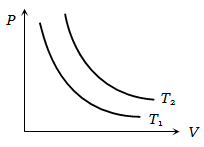1.  ${\mathrm{T}}_{1}>{\mathrm{T}}_{2}$

2. ${\mathrm{T}}_{1}={\mathrm{T}}_{2}$

3. ${\mathrm{T}}_{1}<{\mathrm{T}}_{2}$

4. No inference can be drawn

Subtopic:  Ideal Gas Equation |
To view explanation, please take trial in the course below.
NEET 2023 - Target Batch - Aryan Raj Singh
To view explanation, please take trial in the course below.
NEET 2023 - Target Batch - Aryan Raj Singh
Please attempt this question first.
Launched MCQ Practice Books

Prefer Books for Question Practice? Get NEETprep's Unique MCQ Books with Online Video/Text Solutions via Telegram Bot

The volume V versus temperature T graph for a certain amount of a perfect gas at two pressures  are shown in the figure. Here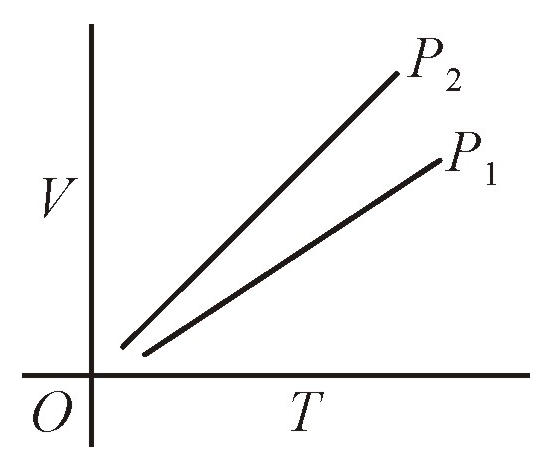1. P1 < P

2. P1 > P2

3. P1 = P2

4.Pressures  can’t be related

Subtopic:  Ideal Gas Equation |
To view explanation, please take trial in the course below.
NEET 2023 - Target Batch - Aryan Raj Singh
To view explanation, please take trial in the course below.
NEET 2023 - Target Batch - Aryan Raj Singh
Please attempt this question first.
Launched MCQ Practice Books

Prefer Books for Question Practice? Get NEETprep's Unique MCQ Books with Online Video/Text Solutions via Telegram Bot

During an experiment, an ideal gas is found to obey an additional law VP2 = constant. The gas is initially at temperature T and volume V. What will be the temperature of the gas when it expands to a volume 2V?

1. $\mathrm{T}\text{'}=\sqrt{4}\mathrm{T}$

2. $\mathrm{T}\text{'}=\sqrt{2}\mathrm{T}$

3. $\mathrm{T}\text{'}=\sqrt{5}\mathrm{T}$

4. $\mathrm{T}\text{'}=\sqrt{6}\mathrm{T}$

Subtopic:  Ideal Gas Equation |
To view explanation, please take trial in the course below.
NEET 2023 - Target Batch - Aryan Raj Singh
To view explanation, please take trial in the course below.
NEET 2023 - Target Batch - Aryan Raj Singh
Please attempt this question first.
Launched MCQ Practice Books

Prefer Books for Question Practice? Get NEETprep's Unique MCQ Books with Online Video/Text Solutions via Telegram Bot

Volume, pressure, and temperature of an ideal gas are V, P, and T respectively. If the mass of its molecule is m, then its density is [k=Boltzmann's constant]
1. mkT
2. $\frac{P}{kT}$
3. $\frac{P}{kTV}$
4. $\frac{Pm}{kT}$

Subtopic:  Ideal Gas Equation |
To view explanation, please take trial in the course below.
NEET 2023 - Target Batch - Aryan Raj Singh
To view explanation, please take trial in the course below.
NEET 2023 - Target Batch - Aryan Raj Singh
Please attempt this question first.
Launched MCQ Practice Books

Prefer Books for Question Practice? Get NEETprep's Unique MCQ Books with Online Video/Text Solutions via Telegram Bot

Which one, of the following, graphs represents the behaviour of an ideal gas at constant temperature?

1.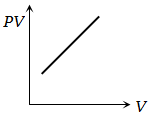2.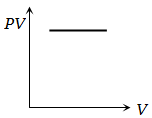3.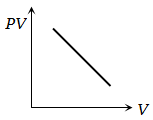4.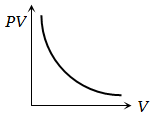Subtopic:  Ideal Gas Equation |
To view explanation, please take trial in the course below.
NEET 2023 - Target Batch - Aryan Raj Singh
To view explanation, please take trial in the course below.
NEET 2023 - Target Batch - Aryan Raj Singh
Please attempt this question first.
Launched MCQ Practice Books

Prefer Books for Question Practice? Get NEETprep's Unique MCQ Books with Online Video/Text Solutions via Telegram Bot

The equation of state for 5g of oxygen at a pressure P and temperature T, when occupying a volume V, will be (where R is the gas constant) :
1. PV = 5 RT
2. PV = (5/2) RT
3. PV = (5/16) RT
4. PV = (5/32) RT

Subtopic:  Ideal Gas Equation |
To view explanation, please take trial in the course below.
NEET 2023 - Target Batch - Aryan Raj Singh
To view explanation, please take trial in the course below.
NEET 2023 - Target Batch - Aryan Raj Singh
Please attempt this question first.
Launched MCQ Practice Books

Prefer Books for Question Practice? Get NEETprep's Unique MCQ Books with Online Video/Text Solutions via Telegram Bot

Two thermally insulated vessels 1 and 2 are filled with air at temperatures  volume  and pressure  respectively. If the valve joining the two vessels is opened, the temperature inside the vessel at equilibrium will be

1. ${\mathrm{T}}_{1}+{\mathrm{T}}_{2}$

2. $\left({\mathrm{T}}_{1}+{\mathrm{T}}_{2}\right)/2$

3. $\frac{{\mathrm{T}}_{1}{\mathrm{T}}_{2}\left({\mathrm{P}}_{1}{\mathrm{V}}_{1}+{\mathrm{P}}_{2}{\mathrm{V}}_{2}\right)}{{\mathrm{P}}_{1}{\mathrm{V}}_{1}{\mathrm{T}}_{2}+{\mathrm{P}}_{2}{\mathrm{V}}_{2}{\mathrm{T}}_{1}}$

4. $\frac{{\mathrm{T}}_{1}{\mathrm{T}}_{2}\left({\mathrm{P}}_{1}{\mathrm{V}}_{1}+{\mathrm{P}}_{2}{\mathrm{V}}_{2}\right)}{{\mathrm{P}}_{1}{\mathrm{V}}_{1}{\mathrm{T}}_{2}+{\mathrm{P}}_{2}{\mathrm{V}}_{2}{\mathrm{T}}_{2}}$

Subtopic:  Ideal Gas Equation |
To view explanation, please take trial in the course below.
NEET 2023 - Target Batch - Aryan Raj Singh
To view explanation, please take trial in the course below.
NEET 2023 - Target Batch - Aryan Raj Singh
Please attempt this question first.
Launched MCQ Practice Books

Prefer Books for Question Practice? Get NEETprep's Unique MCQ Books with Online Video/Text Solutions via Telegram Bot